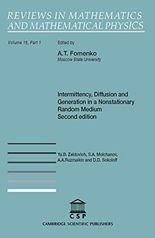• A
• A
• A
• АБB
• АБB
• АБB
• А
• А
• А
• А
• А
Обычная версия сайта
Найдена 1 публикация
Сортировка:
по названию
по годуКнига
Zeldovich Y., Molchanov S., Ruzmaikin A. et al. Vol. 15. Prt. 1. Cambridge: Cambridge Scientific Publishers Ltd, 2015.

This classic survey considers passive scalar and vector transport processes in a random nonstationary medium, which are described by linear parabolic equations. Integration over random paths is used, along with the asymptotic behavior of the product of a large number of independent identically distributed random matrices. The most interesting effect is the appearance of concentrated structures (intermittency) of a smooth initial distribution of the transported quantity. The occurrence of intermittent distributions in the linear problem is due to the fact that the coefficients of the transport equation are stochastic. The intermittency shows itself in the rates of exponential growth of the successive moments (Lyapunov exponents) as the moment number increases. Moment equations are obtained for the scalar and vector, and are used to study temperature evolution and magnetic-field generation in a random fluid flow. These equations are differential in a medium with short time correlations and integral in the general case. The range of application of the diffusion description is analyzed. The behavior of the diffusion coefficients in the case of time reversal is examined. The properties of an individual realization of a scalar and vector are also explained, and a dynamo theorem is given on the exponential growth of the magnetic field in a random flow with renewal.

Добавлено: 22 июня 2016